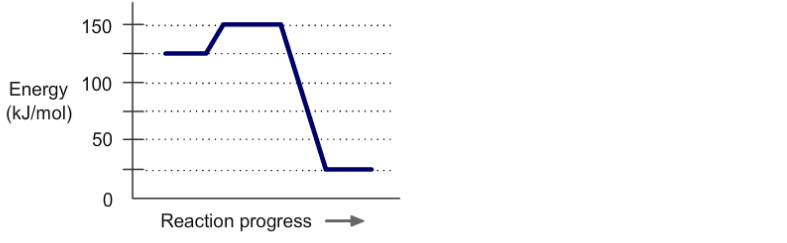# Problem: The diagram represents a spontaneous reaction. Use the diagram to answer the questions below.a. Is the reaction endothermic or exothermic?b. What is the activation energy of the reaction?

###### FREE Expert Solution
99% (496 ratings)
###### FREE Expert Solution

Endothermic - absorbing energy

Exothermic - loss of energy

99% (496 ratings)###### Problem Details

The diagram represents a spontaneous reaction. Use the diagram to answer the questions below.

a. Is the reaction endothermic or exothermic?

b. What is the activation energy of the reaction?What scientific concept do you need to know in order to solve this problem?

Our tutors have indicated that to solve this problem you will need to apply the Energy Diagram concept. If you need more Energy Diagram practice, you can also practice Energy Diagram practice problems.

What is the difficulty of this problem?

Our tutors rated the difficulty ofThe diagram represents a spontaneous reaction. Use the diagr...as medium difficulty.

How long does this problem take to solve?

Our expert Chemistry tutor, Dasha took 2 minutes and 4 seconds to solve this problem. You can follow their steps in the video explanation above.

What professor is this problem relevant for?

Based on our data, we think this problem is relevant for Professor Richter-Addo's class at OU.